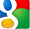# R-example

Created: 2014-05-18 18:31
Updated: 2015-04-03 15:54

# R-example

Programming skills in R

1. `euler16_use_ARRAY.R` The power of array. Use integer array to calculate \$a^n\$ `power(a,n)`.
2. `outer3_use_Vectorized.R` How to use Vectoriz?
3. `char_Split.R` How to use (1) `strsplit` (2) pattern match.
4. `CharMat_SetLocale.R` (1) Uses utf-8 in R-studio; (2) The idea is from a renren post.
5. `Rmd_Tab_Proctoring.Rmd` Shows the way to use css in Rmd.
6. `cchist_PLOT.R ` shows an interesting histogram using other jpg as background. The idea is from internet. As a touhou fan, I used a touhou picture. Check appendix for demo output.
7. `barplot_stackbar.R` gives an example to create complicated bar plot. Check appendix for demo output.
8. cc's deGPS.
9. `yyPLOT_autoadj.R` Will automatically adjust the x-axis.
10. `lock_unlock` shows an example use lock/unlock to control `<<-`,`<-` and the global variables.
11. `Dec2IP_bitwise.R` is an example of bitwise operation which turns the decimal system IP to normal xxx.xxx.xxx.xxx(32bits) version.
12. `Twenty four Game` is an interesting example shows a. Recusive in R b. Relation to Lisp/Scheme

# Appendix

## barplot